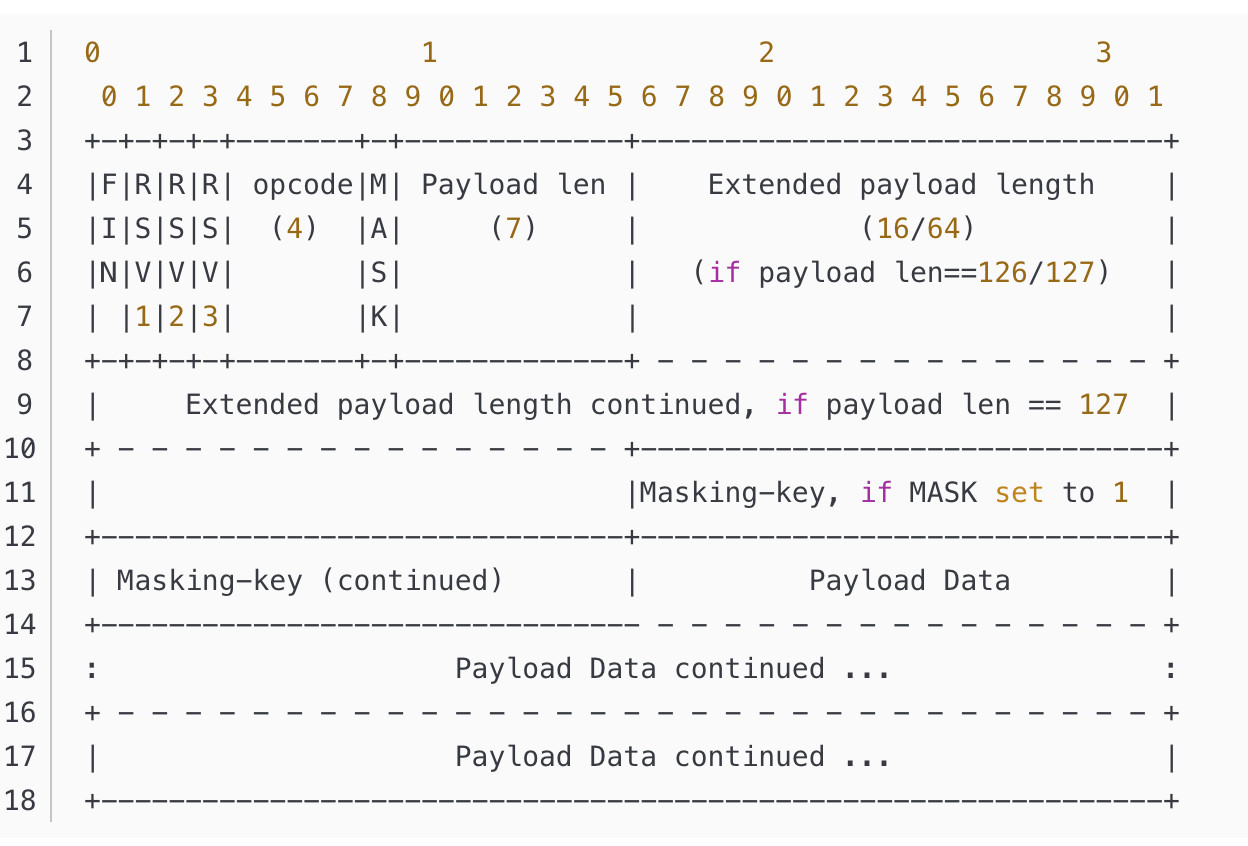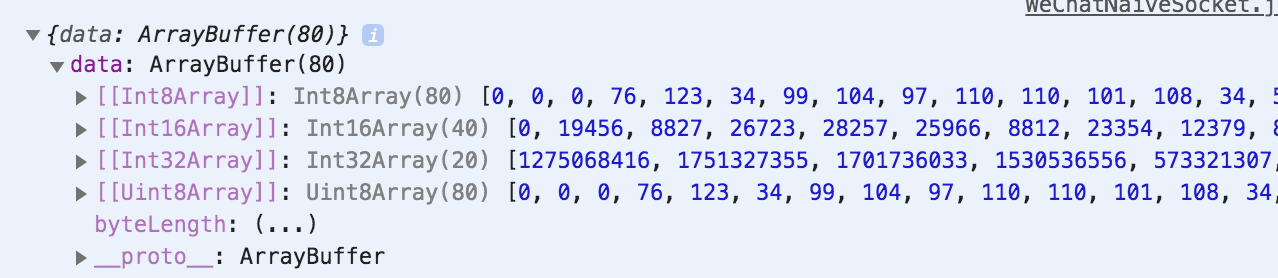WebSocket中的数据类型处理经验分享。

```public static byte[] PackData(string message) {
byte[] contentBytes = null;
byte[] temp = Encoding.UTF8.GetBytes(message);
LogWrite("内容长度：" + temp.Length);
if (temp.Length < 126) {
contentBytes = new byte[temp.Length + 2];
contentBytes = 0x81;
contentBytes = (byte)temp.Length;
Array.Copy(temp, 0, contentBytes, 2, temp.Length);
} else if (temp.Length < 0xFFFF) {
contentBytes = new byte[temp.Length + 4];
contentBytes = 0x81;
contentBytes = 126;
contentBytes = (byte)(temp.Length >>8);
contentBytes = (byte)(temp.Length & 0xFF);
Array.Copy(temp, 0, contentBytes, 4, temp.Length);
} else {
contentBytes = new byte[temp.Length + 10];
contentBytes = 0x81;
contentBytes = 127;
contentBytes = 0;
contentBytes = 0;
contentBytes = 0;
contentBytes = 0;
contentBytes = (byte)(temp.Length >>24);
contentBytes = (byte)(temp.Length >>16);
contentBytes = (byte)(temp.Length >>8);
contentBytes = (byte)(temp.Length & 0xFF);
Array.Copy(temp, 0, contentBytes, 10, temp.Length);
}

return contentBytes;
}
``````0x0表示附加数据帧
0x1表示文本数据帧
0x2表示二进制数据帧
0x3-7暂时无定义，为以后的非控制帧保留
0x8表示连接关闭
0x9表示ping
0xA表示pong
0xB-F暂时无定义，为以后的控制帧保留
```2 回复